Time Travel Research Center © 1998 Cetin BAL - GSM:+90  05366063183 -Turkey/DenizliPrinciples of gravity manipulation and “Stargate”-technology via Quantum Vacuum

in:   Journal of Theoretics, Vol. 4, No. 4, August 2002 (preprint)

Carlos Calvet

Francisco Corbera no. 15, E-08360 Canet de Mar (Barcelona), Spain

Düzenleme: Çetin BAL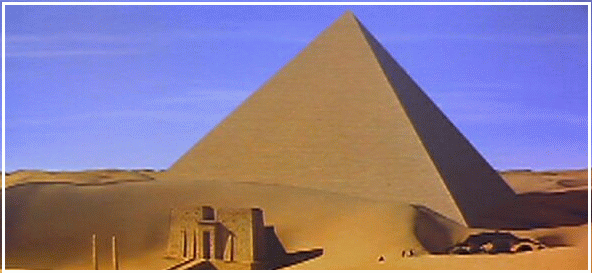Abstract

By expressing natural constants in terms of Planck units, we found that Universal Gravitation Constant is the inverse of vacuum density matter-equivalent and the square of Planck time, being the former equal to Planck mass divided by Planck volume. The corresponding new equation of gravitation reveals that gravitation can be manipulated via vacuum energy. “Weak gravity shielding experiments” are interpreted as the likely result of uploading photons from E.M.-fields to vacuum by using superconductor arrangements.

In addition, from Coulomb’s constant, we derived the “Planck charge” and the corresponding density of virtual vacuum pairs. Planck charge can be understood as 6 strings existing in a Planck volume, equivalent to the 6 dimensions supposed to exist at string level. By combining both models, it results that quantum vacuum is a six-dimensional space that links elementary particles through the “other side”, thus allowing entangled particles to display well-known non-locality properties. In consequence, strings are not mutually isolated Kaluza-Klein spaces, but all strings are interconnected via quantum vacuum.Matter waves produced by Bose-Einstein condensation are therefore the likely result of fusing supercold Kaluza-Klein spaces together, and represent an “event horizon” that marks a path between spacetime and quantum vacuum. By condensing large amounts of solid matter, so-called “Stargate” technology could be in reach within several decades or less.

Key words: Gravitation, zero point energy, inertia, electrogravity, Coulomb’s Constant, Planck charge, virtual pairs, quantum vacuum, event horizonIntroduction

In two articles , , we derived respectively the Universal Gravitation Constant (G) from Planck units and demonstrated that, spacetime and quantum vacuum (QV), are two different spaces.

Because Newton’s equation of gravitation has two components (a constant [G] and a variable mass component [m1m2/d2]), these two components can be treated separately, since they are independent. In consequence, gravitation can be considered a combined force, consisting of G (as demonstrated, a QV function) and conventional “mass attraction”, the latter produced by gravity fields and/or spacetime geometry according to the corresponding theories. This principle of independence allowed us to calculate inertia for mutually attracting and/or otherwise accelerated bodies, finding that inertia has a very high value, so that its fundamental effect is probably that of marking a clear distinction between fermions (matter) and photons (light), the latter not being affected by inertia.

While “mass attraction” depends only of mutually attracting masses and their relative position or distance, G (a so-called, “non-derivable constant”) resulted to be effectively derivable and is the exact inverse of “vacuum mass density equivalent” (5.156x1096 kg/m3) (Planck mass divided by Planck volume) and the square of Planck time, the former having already been predicted before as a approximate value, e.g. in . The new equation of gravitation resulting from substituting G by Planck units reveals that vacuum density (analogous to Zero Point Radiation [ZPR]) affects gravity inversely. This means that, if we managed to increase ZPR, gravity would decrease and vice-versa. (In fact, the very small value of G [6.673x10-11 m3kg-1s-2] already suggests that there is “something” that weakens mass attraction. We found in  that this “something” is ZPR).

The above findings, in combination with so-called "weak gravitation shielding experiments”, all related to , , reveal that gravity can be effectively manipulated via QV by using superconductor arrangements.

In our second article , we derived the (generally unknown or as “electron charge”

misunderstood) “Planck charge” (1.876x10-18 C) from Coulomb’s Constant (C), corresponding to the charge existing in a QV-Planck volume. By comparing the corresponding QV lepton/photon ratio with the almost equivalent baryon/photon ratio predicted for spacetime by Standard Model, we found that the former is about 12 orders of magnitude lower, allowing to state under any circumstance that spacetime and QV are two different spaces (even if we corrected somehow the corresponding ratios, the difference would be still too large to be merely insignificant).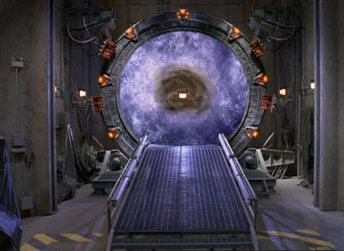These findings allow to determine that spacetime is mainly a space full of neutral matter and much light, while QV is mainly a space full of strong radiation and some charges (virtual pairs), so that both universes are effectively principally incompatible and ought to exist therefore - even from a theoretical point of view - as separated spaces in the universe.

By dividing Planck charge by the charge of the electron (1.6022x10-19 C), we obtain an average density of 11.71 leptons per Planck volume, which per definition correspond to the well known virtual electron-positron pairs that create and anihilate mutually in vacuum. At the very moment of their mutual interaction, these approx. 12 entire leptons correspond to 6 particles or strings and this number is coincident with the 6 Kaluza-Klein dimensions, attributed to strings.This coincidence allows to establish a direct link between strings and QV as already suggested by The California Institute for Physics and Astrophysics in its homepage (“it now appears that quantum field theory may be the low energy limit of superstring theory”) and suggests that QV is the space where strings are physically located. This model would immediately explain the effect known as “quantum non-locality”, since strings (in so-called “entangled particles”) would interact instantly via QV in a time that is zero for spacetime observers, since QV disposes of a different time frame than spacetime because of its different number of dimensions, and QV-time has therefore no meaning to us.Gravity manipulation

As already shown in , vacuum mass-density equivalent can be understood per definition as a Planck mass existing in a Planck volume: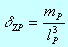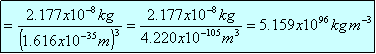(1)

where: dZP = vacuum mass-density equivalent, mP = Planck mass, and l3P = VP (Planck volume) (see also  for a parallel derivation).

By substituting G by the corresponding Planck units, we get:(2)

and substituting: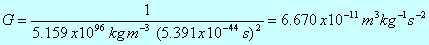(3)

which is equal (up to the rounded decimals) to the normal value of G (6.673x10-11 m3kg-1s-2) and demonstrates that G corresponds effectively to function (2), i.e., that G is a QV-function.

In consequence, Newton’s equation of gravitation adopts the following form by substituting G by eq. (2):(4)

In this equation, we call the above-mentioned independent right component “mass attraction” because it depends only on masses and their relative position. According to the “cause-effect principle”, the left component (G) can be considered “vacuum reaction” to gravitation since matter is obviously the origin of any gravitation. (A similar vacuum reaction to accelerated matter is known as Davies-Unruh effect (, ) and demonstrates that vacuum effectively reacts to the presence of matter. In addition, since in (4), dZP represents vacuum mass-density equivalent, reaction to gravitation is a vacuum effect).The first we observe in (4) is that gravity is inversely proportional to vacuum energy (dZP). In consequence, if we manipulated vacuum energy, we would parallel be manipulating gravity.

Further, if QV did not exist, ZPR would be zero and according to (4), gravitational force would be infinite. And opposite, if ZPR was infinite, gravity would be zero. In , we already mentioned a herewith related case with regard to the extreme high temperature of the solar corona (up to 2x106°C) with respect to the photosphere or surface of the sun (only 5,500°C). Probably, the very dense solar photon stream produces “holes” in the fabric of spacetime, so that ZPR emerges from QV and heats up the solar corona. In consequence, we predict that in the solar corona, gravity could be weaker than normal.

To understand the nature of QV and why ZPR is able to reduce gravity, we make the following experiment of thought:

Imagine a flat universe (sheet) located in spacetime (our frame). Any spacetime radiation that crosses the sheet, will produce effects in the sheet, but will not remain there. Eventual inhabitants of the sheet (flatlanders) will notice radiation effects, but will see no radiation. Analogous happens in our universe: Vacuum radiation that crosses spacetime, produces Casimir-like effects, but cannot be seen nor detected because of its alien location.

Since QV is a 6-D space  that surrounds 4-D spacetime completely due to its natural superior extension, spacetime matter is completely surrounded by ZPR. If an object is moving uniformly or at rest, ZPR will be the same on any surface. But as soon as the object accelerates, a Doppler-effect takes place (see also  for analogous explanation), so that ZPR becomes more dense in the direction of movement and less dense behind the object. This produces a higher ZPR pressure in the opposite direction of movement, so that an effective “vacuum reaction” takes place, with the consequence that the initial acceleration is reduced. This effect is commonly known as “inertia” and per definition also somehow related to the much weaker Davies-Unruh effect.

This means that vacuum reacts to acceleration by opposing ZPR-borne inertia.

In the case of static bodies, ZPR produces a homogenous radiation pressure, so that no neat vacuum reaction or inertia takes place. But if we managed to increase ZPR, according to (4), we would induce vacuum reaction artificially and gravitation would therefore weaken in a parallel extent. In consequence, not only acceleration is able to produce vacuum reaction (Davies-Unruh, inertia), but also any phenomenon that affects vacuum density.

This can be understood as that vacuum reaction happens in the opposite direction to the existing gravitational fields. Through Newtons equation of motion, F=m·a, any body subjected to a field of force (in this case, gravitation), is also subjected to a potential acceleration toward attracting bodies. In consequence, vacuum reaction will produce a reaction force via ZPR pressure that is opposed to the main direction of the corresponding gravitational fields, with the final result that, even bodies in a stiff gravitational system are subjected to a neat ZPR reaction force opposite to the direction of the field (although not to inertia, since they are not accelerated. In consequence, inertia and vacuum reaction to a force differ in stiff systems and are not exactly the same).

With the above model in mind, we interpret Podkletnov’s famous "weak gravitation shielding experiment” as the obvious result of QV manipulation with the aid of E.M. fields, produced by a spinning superconductor. In effect, it seems that the fields induced by the superconductor and/or the coils used for levitation, did increase local ZPR, probably by uploading photons from E.M. fields to QV. At that point, according to eq. (4), the higher ZPR should have reduced gravity, which was effectively observed by Podkletnov  and later also by Li  and several others.

This means that, it was not the superconductor itself that affected gravity, but the resulting E.M. fields. Probably, a flow of photons from spacetime to QV takes place if strong E.M. fields interact mutually, e.g. by making one field rotate inside another as in . The resulting “friction” between both fields could provide the necessary energy to upload photons from spacetime fields to QV, thus increasing local ZPR and reducing gravity according to eq. (4).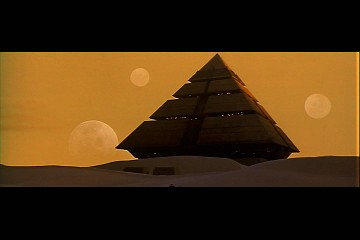This principle suggests further that ZPR is not uniform in the universe, but that there could be many local phenomena that increased or decreased vacuum energy, making therefore decrease or increase respectively local gravity. The universe can be in this sense understood at least as a giant gravity device via QV. The expansion of the universe could be so, at least in part, interpreted as the result of ZPR repulsion on matter. Emerging ZPR in intergalactic space could explain intergalactic voids as well as global dispersion of the universe.

To alter ZPR, we could use any kind of device or system, able to create a photon flow from QV to spacetime and/or vice-versa. It seems at this stage easier to weaken gravity by making interfere spinning intense E.M. fields mutually or flooding tiny spaces with large amounts of photons , than to increase gravity by contrary means.

Anyhow, to increase gravity, it is necessary to extract photons from QV. May be black holes, neutron stars and other dense objects do produce or absorb some radiation of this kind. By compressing supercold matter (Bose-Einstein condensate), instead of obtaining fusion processes as in a conventional hydrogen bomb, we already suggested in  that it should be able to create superdense matter. Arranging this matter in devices, it should be able to download photons from QV in order to increase local gravity. A spacecraft equipped with a combination of several of the above-mentioned technologies, should be able to navigate without the need of any propulsion, at least in the proximities of celestial objects.

All the above-mentioned are methods dedicated to alter locally the Universal Gravitation Constant “G”. But the right component of eq. (4), consisting of “mass attraction”, is the phenomenon that, in principle, induces vacuum reaction (left component). This means that, apart from vacuum reaction, masses attract mutually by some sort of “gravitational field” or “spacetime geometry” as predicted respectively by quantum field theories and general relativity. Therefore, another way to alter gravity, would be manipulating such fields or geometries - a theme that is out of the scope of this paper.

The novelty of eq. (4) is that it demonstrates that gravity is not really subjected to any kind of constant, but that it is the result of the balance between gravitational attraction and vacuum repulsion on spacetime objects subjected to ZPR. Nature has probably provided this apparently complex system to allow a more stable universe, not subjected to unbiased and/or infinite field intensities and/or accelerations . A universe without QV component would probably be a very chaotic place if it had ever existed at all.“Stargate”-technology

Following an analogous method as once Max Planck when he derived Planck units from natural constants, and expressing Coulomb’s Constant (C = 8.988x109 Nm2/C2) in term of Planck units, in  we found the charge contained in a Planck volume (Planck charge):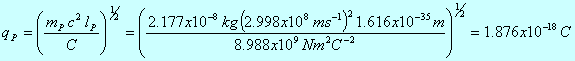(5)

This charge, divided by the charge of the electron (1.6022x10-19 C), renders the amount of 11.71 leptons per Planck volume, corresponding to well-known virtual electron-positron pairs.

Since Coulomb’s Constant is a function of vacuum permittivity (e0) and/or permeability (µ0), Planck charge can be interpreted as the charge of virtual pairs existing in vacuum. In consequence, any vacuum Planck volume contains 11.71 virtual leptons. 11.71 is a mean value, which means that there can be 10, 11, 12, 13 etc. leptons at any time in a Planck volume, but that the mean is 12 entire leptons, because this is the entire figure that most resembles 11.71.

12 entire leptons correspond to 6 virtual pairs. It is known that any pair anihilates mutually and produces a gamma particle pair, which again anihilates mutually and renders an electron-positron pair in an almost immediate and endless sequence inside a much confined space. At the very moment of their mutual interaction, 12 leptons/gamma particles fuse and represent therefore approx. the volume of 6 particles/strings. Sine any string is considered to be unidimensional and of the Planck length, 6 strings would fill up the space corresponding to a 6 dimensional Planck volume.

Since the above-mentioned 6 virtual pairs are contained in a Planck volume that according to Coulomb’s Constant is a vacuum volume, it results that vacuum is a 6-dimensional space. (In fact, 6 one-dimensional strings concentrated on one spot, have per definition the extension of a 6-dimensional Planck volume).

In , we also showed that, by comparing the fermion/photon ratio of spacetime and QV, it results that QV contains 12 orders of magnitude more photons than spacetime. This enormous difference between both principally infinite macroscopic spaces, together with the above 6 dimensions found to exist in QV, demonstrate that QV and spacetime are different spaces, i.e. that they are not the same space.

Further, it is generally known from string theory that particles consist of 10-dimensional strings. These 10 dimensions correspond to 4 outside spacetime dimensions (the outer shell of the corresponding particle) and 6 curled dimensions inside string environment that are supposed to be a relict of Big Bang, so that the original 10 dimensional particle that exploded at the beginning of the universe rendered a large amount of smaller particles that carry inside curled 6-dimensional (Kaluza-Klein) universes of about the Planck size.

The 6 dimensions attributed to string environment coincide exactly with the number of 6 dimensions we found to exist in QV. In consequence, QV can be considered the medium, in which strings are located, so that any Kaluza-Klein universe that surrounds a particular string is connected to QV through a path to the “other side” that is not directly accessible through spacetime at normal conditions.

On the other hand, it is generally known that entangled particles do display so-called “non-locality”. That is the ability of particles like bosons to get synchronized at very large distances and is interpreted as the immediate interchange of information in a time that is zero for spacetime observers (see for ex.  for additional explanation).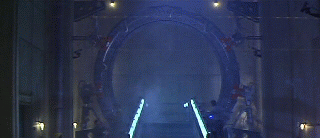With our model of strings surrounded by 6-dimensional QV, particle non-locality can be easily understood as the ability of elementary particles to interact through QV. As above-mentioned, each particle can be understood as consisting of an outer 4-dimensional shell (spacetime side) and an inner 6-dimensional string (QV side). While the outer shell would be responsible e.g. for conventional photon interchange via spacetime, the inner shell would be responsible for mutual “communication” as known to exist with entangled particles. Since communication in 6-dimensional QV happens in a time frame beyond 4-dimensional spacetime, any QV interaction happens in a time that has no meaning for spacetime observers, i.e., the time needed by two entangled particles to interact results to be zero for us and for all of our spacetime devices.

As the result, our universe can be understood as consisting of two different spaces (spacetime and QV) linked together by elementary particles, so that the outer shell of any particle corresponds to the well known 4-dimensional particle, while the inner side is built up by a string surrounded by a local portion of 6-dimensional QV. Generally speaking, any particle can be considered as being a small window to QV.

In the past, there had been no possibility to fuse these tiny windows in order to create a window, large enough to be used e.g. as a path to the “other side”, but the recent discovering of so-called “Bose-Einstein condensation” (BEC) makes it now possible. In fact, at a temperature of only one-billionth degree Kelvin, atoms turn into matter waves and acquire quantum properties. This means that BEC atoms behave like bosons and that they are able to overlap and to produce one single wave, made out of all the small waves that constitute the corresponding atoms.

By fusing supercold atoms into a matter wave, what we are really doing, is eliminating the outer 4-dimensional shell of elementary particles, thus allowing 6-dimensional QV to emerge and to provide quantum properties to the wave, which are per definition usually confined inside QV. Since at BEC, a large amount of particles fuses into one single wave, all Kaluza-Klein universes of the corresponding strings do fuse too and build a path to QV that could be theoretically enlarged and used technologically.

A logic question that remains is, how it is possible to put two spaces together that have each one a different number of dimensions (4 vs. 6)?

Nature has provided the solution to this question through the giant matter wave that results from the fusing of individual atoms. This wave can be understood as an “event horizon” at the boarder between spacetime and QV, and resembles in some way the event horizon of a black hole. In this sense, a matter wave (in opposition to a light wave) acts as a large window or path between spacetime and QV. (Light can be understood as consisting of strings that are not connected to QV and that simply “ride” upon spacetime fabrics. This “simple” difference would mark the difference between matter and energy).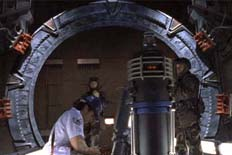In order a large BEC window to be used as a path for devices or probes to explore the other side, we ought to create a BEC that is huge enough for this purpose. Current BEC are very fragile and consist only of a diluted cloud of dispersed atoms. A technically useful BEC-window or “Stargate” should consist of a large dense mass of BEC condensed solid matter, large enough, to provide sufficient space and dynamism for probes coming and going. To achieve this goal, we must improve the current techniques that allow creation of BEC (magnetic traps, laser light spotting, etc.) and use more powerful ones, such as matter blocks submerged in a circuit filled with superfluid (e.g. He-4). (Even if superfluids are no perfect BECs, a counter-flow of such fluids will produce a constant energy loss in matter blocks submerged in that flow, probably until BEC-temperature. In consequence, even with relatively simple technologies, it might be possible to produce large solid BECs, by just using the right technique).

Once a window large enough is achieved, we would dispose of a path to enter macroscopic QV. At this stage, there are two possibilities of using such a Stargate: -

- 1. By using one single window, we would dispose of a path from spacetime to QV and vice-versa.

- 2. By using two windows, we could eventually dispose of a path from one window to the other, without having to cross physically QV.

In the following, we will briefly discuss both possibilities: -

- 1. Vacuum properties as Planck frequency (?P) reveal that QV is a macroscopic space, full of very strong ZPR, even in the range of gamma particles, as to be expected from the content of mutually anihilating virtual pairs and from the mean radiation frequency ((?P)1/2 = approx. 1021 Hz). In consequence, any probe or body that entered QV, would be exposed at least to a severe gamma-ray shower that would threaten its integrity. We therefore suggest considering this possibility as a means to send and to receive signals as long as we do not dispose of more details.

- 2. In order to use two windows, they should be synchronized and/or connected in some way in order to achieve parallel functioning. This could be eventually achieved by dividing a BEC block into two smaller blocks, while using each block for a different window. In this case, synchronization would be analogous as with entangled particles. By disposing of 2 windows, each one could be used as entrance and/or exit.

In any case, any physical trajectory through QV must be considered as being very dangerous for materials and entities exposed. The QV effects, we do know (Casimir effect, etc.) do not reveal the deathly nature of vacuum gamma rays and other radiation of still unknown nature, responsible for those practically insignificant spacetime effects. It is unknown what kind of radiation exists above gamma range. One possibility is that ZPR contains also gluons that would make up the range from 1021 - 1043 Hz. In this case, we would be faced with a complete new physics of free gluons in a 6-dimensional space - a situation analogous as submerging ourselves inside a quantum.

Despite any difficulty that could exist in producing the above-mentioned technologies, we are confident that they are in reach within the following 25 years or even earlier if the idea is carried out willingly.Comment:

It has been criticized that we did not use relativistic approaches to the corresponding equations. But this criticism is senseless because at Planck level, there is no relativity. Max Planck derived his fundamental units directly from natural constants without using any relativistic approach. Natural constants provide information about QV directly. In this sense, the speed of light is the exact value of Planck length divided by Planck time and vacuum energy corresponds exactly to Planck mass divided by Planck volume. If we introduced relativity into Planck level, we would be converting fundamental reality into an antropic point of view. Relativity probably takes place somewhere between Planck world and normal level. Neither Planck level nor cosmological level seem to be subjected to any sort of relativity - they are fundamental!

References

       Carlos Calvet, “Gravitation and inertia as a consequence of Quantum Vacuum Energy”, Journal of Theoretics, Vol. 4, No. 2, April 2002 (preprint)

       Carlos Calvet, “About the quantum vacuum lepton/photon ratio”, Journal of Theoretics, Vol. 4, No. 2, April 2002 (preprint)

       H.E. Puthoff, “The Energetic Vacuum: Implications For Energy Research, Speculations in Science and Technology”, Vol. 13, No. 4, pp. 247-257 (1990)

       E.E. Podkletnov (Moscow Chem. Scientific. Ctr.), "Weak Gravitation Shielding Properties of Composite Bulk YBa2Cu3O7-x Superconductor Below 70°K under E.M. Field," Univ. Cincinnati Engineering, report # MSU-chem 95, abstract cond-mat/9701074, 19 pp. (1997)

       N. Li, D. Noever, T. Robertson, R. Koczor and W. Brantley, “Static Test for A Gravitational Force Coupled to Type II YBCO Superconductors”, Physica C, Vol. 281, pp. 260-267 (1997)

       S.K. Lamoreux, “Demonstration of the Casimir force in the 0.6 to 6 mm range”, Phys. Rev. Lett., Vol. 78, No. 1, pp. 5-8 (1997)

       P.W. Milonni, R.J. Cook, & M.E. Goggin, “Radiation pressure from the vacuum: Physical interpretation of the Casimir force”, Phys. Rev. A, Vol. 38, No. 3, pp. 1621-1623 (1988)

       H.G.B. Casimir, “On the attraction between two perfectly conducting plates”, Proc. Kon. Ned. Akad. van Weten., Vol. 51, No. 7, pp. 793-796 (1948)

       P.C.W. Davies, “Scalar particle production in Schwarzschild and Rindler metrics”, J. Phys. A, Vol. 8, p. 609 (1975)

     W.G. Unruh, “Notes on black-hole evaporation”, Phys. Rev. D, Vol. 21, p. 2137 (1980)

     Carlos Calvet, “Effects and Evidence of the Background Field”, Journal of Theoretics, Vol.2, No.4, Aug 2000 (preprint)

     Carlos Calvet, “Evidence for the Existence of 5 Real Spatial Dimensions in Quantum Vacuum - Scale of Quantum Temperatures Below Zero Kelvin”, Journal of Theoretics, Vol.3, No.1, Feb. 2001 (preprint)

     Raymond Y. Chiao, Paul G. Kwiat, Aephraim M. Steinberg, "Quantum non-locality in Two-Photon Experiments at Berkeley" (International Workshop on Laser and Quantum Optics, Nathiagali, Pakistan, 9-14 July 1994) in Quantum and Semiclassical Optics 7, 259-78 (was preprint quant-ph/950101)

Hiçbir yazı/ resim  izinsiz olarak kullanılamaz!!  Telif hakları uyarınca bu bir suçtur..! Tüm hakları Çetin BAL' a aittir. Kaynak gösterilmek şartıyla  siteden alıntı yapılabilir.

© 1998 Cetin BAL - GSM:+90  05366063183 - Turkiye / Denizli

Ana Sayfa /Index /Roket bilimi / E-Mail /Astronomy/

Time Travel Technology /UFO Galerisi  /UFO Technology/

Kuantum Teleportation /Kuantum Fizigi /Uçaklar(Aeroplane)